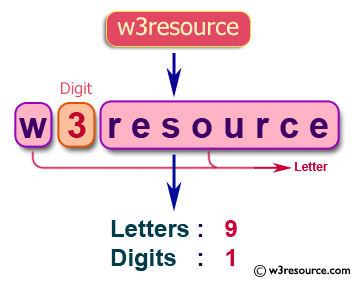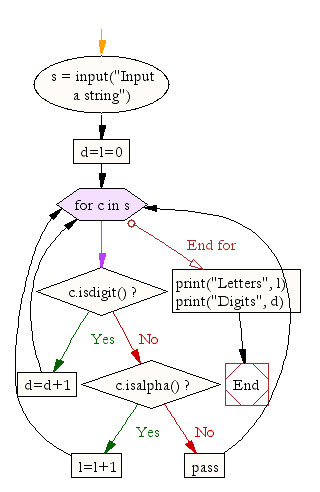﻿ Python Exercise: Calculate the number of digits and letters in a string - w3resource# Python Exercise: Calculate the number of digits and letters in a string

## Python Conditional: Exercise-14 with Solution

Write a Python program that accepts a string and calculate the number of digits and letters.

Pictorial Presentation:Sample Solution:

Python Code:

``````s = input("Input a string")
d=l=0
for c in s:
if c.isdigit():
d=d+1
elif c.isalpha():
l=l+1
else:
pass
print("Letters", l)
print("Digits", d)
```
```

Sample Output:

```Input a string W3resource
Letters 9
Digits 1
```

Flowchart:## Visualize Python code execution:

The following tool visualize what the computer is doing step-by-step as it executes the said program:

Python Code Editor:

Have another way to solve this solution? Contribute your code (and comments) through Disqus.

What is the difficulty level of this exercise?

Test your Python skills with w3resource's quiz

﻿

## Python: Tips of the Day

Returns a list with n elements removed from the beginning

Example:

```def tips_take(itr, n = 1):
return itr[:n]
print(tips_take([1, 2, 3], 5))
print(tips_take([1, 2, 3], 0))
```

Output:

```[1, 2, 3]
[]
```